• 目标： ...对于图像，2D离散傅里叶变换（DFT）用于找到频域。称为快速傅里叶变换（FFT）的快速算法用于计算DFT。有关这些的详细信息可以在任何图像处理或信号处理教科书中找到。 对于正弦信号，x（t...
目标：

使用OpenCV查找图像的傅立叶变换
使用Numpy中提供的FFT函数
傅立叶变换的一些应用
我们将看到以下函数：cv.dft()，cv.idft()等

理论
傅立叶变换用于分析各种滤波器的频率特性。对于图像，2D离散傅里叶变换（DFT）用于找到频域。称为快速傅里叶变换（FFT）的快速算法用于计算DFT。有关这些的详细信息可以在任何图像处理或信号处理教科书中找到。
对于正弦信号，x（t）= Asin（2πft），我们可以说f是信号的频率，如果采用其频域，我们可以看到f处的尖峰。如果对信号进行采样以形成离散信号，则我们得到相同的频域，但在[-π，π]或[0,2π]（或对于N点DFT的[0，N]）范围内是周期性的。你可以将图像视为在两个方向上采样的信号。因此，在X和Y方向上进行傅里叶变换可以得到图像的频率表示。
更直观地说，对于正弦信号，如果幅度在短时间内变化如此之快，则可以说它是高频信号。如果变化缓慢，则为低频信号。你可以将相同的想法扩展到图像。幅度在图像中的幅度变化很大？在边缘点，或噪音。我们可以说，边缘和噪声是图像中的高频内容。如果幅度没有太大变化，则它是低频分量。 （一些链接被添加到Additional Resources_，它通过示例直观地解释了频率变换）。
现在我们将看到如何找到傅立叶变换。
Numpy中的傅里叶变换
首先，我们将看到如何使用Numpy找到傅立叶变换。Numpy有一个FFT包来做到这一点。np.fft.fft2()为我们提供了一个复杂数组的频率变换。它的第一个参数是输入图像，它是灰度。第二个参数是可选的，它决定了输出数组的大小。如果它大于输入图像的大小，则在计算FFT之前用零填充输入图像。如果小于输入图像，则将裁剪输入图像。如果没有传递参数，则输出数组大小将与输入相同。
现在，一旦得到结果，零频率分量（DC分量）将位于左上角。如果要将其置于中心位置，则需要在两个方向上将结果移动$\frac{N}{2}$。这只是通过函数np.fft.fftshift()完成的。找到频率变换后，你可以找到幅度谱。
import cv2 as cv
import numpy as np
from matplotlib import pyplot as plt

img = cv.imread('messi5.jpg',0)
f = np.fft.fft2(img)
fshift = np.fft.fftshift(f)
magnitude_spectrum = 20*np.log(np.abs(fshift))
plt.subplot(121),plt.imshow(img, cmap = 'gray')
plt.title('Input Image'), plt.xticks([]), plt.yticks([])
plt.subplot(122),plt.imshow(magnitude_spectrum, cmap = 'gray')
plt.title('Magnitude Spectrum'), plt.xticks([]), plt.yticks([])
plt.show()

窗口将如下图显示：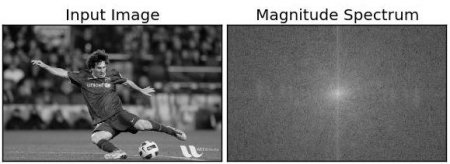请注意，你可以在中心看到更多更白的区域，显示低频内容更多。
所以你找到了频率变换现在你可以在频域做一些操作，比如高通滤波和重建图像，即找到逆DFT。 为此，你只需通过使用尺寸为60x60的矩形窗口进行遮罩来移除低频。 然后使用np.fft.ifftshift()应用反向移位，以便DC组件再次出现在左上角。 然后使用np.ifft2()函数找到逆FFT。 结果再次是一个复杂的数字。 你可以采取它的绝对价值。
rows, cols = img.shape
crow,ccol = rows/2 , cols/2
fshift[crow-30:crow+30, ccol-30:ccol+30] = 0
f_ishift = np.fft.ifftshift(fshift)
img_back = np.fft.ifft2(f_ishift)
img_back = np.abs(img_back)
plt.subplot(131),plt.imshow(img, cmap = 'gray')
plt.title('Input Image'), plt.xticks([]), plt.yticks([])
plt.subplot(132),plt.imshow(img_back, cmap = 'gray')
plt.title('Image after HPF'), plt.xticks([]), plt.yticks([])
plt.subplot(133),plt.imshow(img_back)
plt.title('Result in JET'), plt.xticks([]), plt.yticks([])
plt.show()

窗口将如下图显示：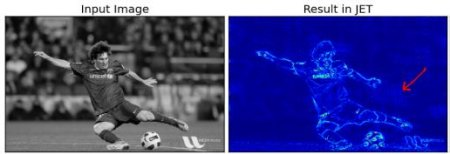结果显示高通滤波是边缘检测操作。这是我们在Image Gradients章节中看到的。这也表明大多数图像数据存在于光谱的低频区域。无论如何，我们已经看到如何在Numpy中找到DFT，IDFT等。现在让我们看看如何在OpenCV中完成它。
如果你仔细观察结果，特别是JET颜色的最后一个图像，你可以看到一些文物（我用红色箭头标记的一个实例）。它在那里显示出一些类似波纹的结构，它被称为振铃效应。它是由我们用于遮蔽的矩形窗口引起的。此蒙版转换为sinc形状，这会导致此问题。因此矩形窗口不用于过滤。更好的选择是高斯Windows。
OpenCV中的傅里叶变换
OpenCV为此提供了cv.dft()和cv.idft()函数。它返回与之前相同的结果，但有两个通道。第一个通道将具有结果的实部，第二个通道将具有结果的虚部。输入图像应首先转换为np.float32。我们将看到如何做到这一点。
import cv2 as cv
from matplotlib import pyplot as plt
img = cv.imread('messi5.jpg',0)
dft = cv.dft(np.float32(img),flags = cv.DFT_COMPLEX_OUTPUT)
dft_shift = np.fft.fftshift(dft)
magnitude_spectrum = 20*np.log(cv.magnitude(dft_shift[:,:,0],dft_shift[:,:,1]))
plt.subplot(121),plt.imshow(img, cmap = 'gray')
plt.title('Input Image'), plt.xticks([]), plt.yticks([])
plt.subplot(122),plt.imshow(magnitude_spectrum, cmap = 'gray')
plt.title('Magnitude Spectrum'), plt.xticks([]), plt.yticks([])
plt.show()


注意：你还可以使用cv.cartToPolar（），它可以一次性返回幅度和相位

所以，现在我们必须进行逆DFT。 在之前的会话中，我们创建了一个HPF，这次我们将看到如何去除图像中的高频内容，即我们将LPF应用于图像。 它实际上模糊了图像。 为此，我们首先在低频处创建具有高值（1）的掩模，即我们传递LF内容，并且在HF区域传递0。
rows, cols = img.shape
crow,ccol = rows/2 , cols/2
# create a mask first, center square is 1, remaining all zeros
mask = np.zeros((rows,cols,2),np.uint8)
mask[crow-30:crow+30, ccol-30:ccol+30] = 1
# apply mask and inverse DFT
fshift = dft_shift*mask
f_ishift = np.fft.ifftshift(fshift)
img_back = cv.idft(f_ishift)
img_back = cv.magnitude(img_back[:,:,0],img_back[:,:,1])
plt.subplot(121),plt.imshow(img, cmap = 'gray')
plt.title('Input Image'), plt.xticks([]), plt.yticks([])
plt.subplot(122),plt.imshow(img_back, cmap = 'gray')
plt.title('Magnitude Spectrum'), plt.xticks([]), plt.yticks([])
plt.show()

窗口将如下图显示：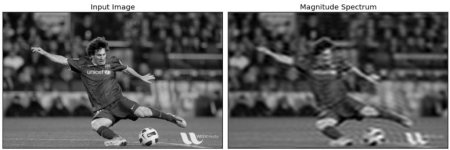注意：像往常一样，OpenCV函数cv.dft()和cv.idft()比Numpy函数更快。 但是Numpy功能更加用户友好。 有关性能问题的更多详细信息，请参阅以下部分。

DFT的性能优化
对于某些阵列大小，DFT计算的性能更好。 当阵列大小为2的幂时，它是最快的。 尺寸为2，3和5的乘积的阵列也可以非常有效地处理。 因此，如果你担心代码的性能，可以在找到DFT之前将数组的大小修改为任何最佳大小（通过填充零）。 对于OpenCV，你必须手动填充零。 但对于Numpy，你可以指定FFT计算的新大小，它会自动为你填充零。
那么我们如何找到这个最佳尺寸？ OpenCV为此提供了一个函数cv.getOptimalDFTSize（）。 它适用于cv.dft（）和np.fft.fft2（）。 让我们使用IPython magic命令timeit检查它们的性能。
In : img = cv.imread('messi5.jpg',0)
In : rows,cols = img.shape
In : print("{} {}".format(rows,cols))
342 548
In : nrows = cv.getOptimalDFTSize(rows)
In : ncols = cv.getOptimalDFTSize(cols)
In : print("{} {}".format(nrows,ncols))
360 576

看，大小（342,548）被修改为（360,576）。 现在让我们用零填充它（对于OpenCV）并找到它们的DFT计算性能。 你可以通过创建一个新的大零数组并将数据复制到它，或使用cv.copyMakeBorder（）来实现。
nimg = np.zeros((nrows,ncols))
nimg[:rows,:cols] = img

或者
right = ncols - cols
bottom = nrows - rows
bordertype = cv.BORDER_CONSTANT #just to avoid line breakup in PDF file
nimg = cv.copyMakeBorder(img,0,bottom,0,right,bordertype, value = 0)

现在我们计算Numpy函数的DFT性能比较：
In : %timeit fft1 = np.fft.fft2(img)
10 loops, best of 3: 40.9 ms per loop
In : %timeit fft2 = np.fft.fft2(img,[nrows,ncols])
100 loops, best of 3: 10.4 ms per loop

它显示了4倍的加速。现在我们将尝试使用OpenCV函数。
In : %timeit dft1= cv.dft(np.float32(img),flags=cv.DFT_COMPLEX_OUTPUT)
100 loops, best of 3: 13.5 ms per loop
In : %timeit dft2= cv.dft(np.float32(nimg),flags=cv.DFT_COMPLEX_OUTPUT)
100 loops, best of 3: 3.11 ms per loop

它还显示了4倍的加速。 你还可以看到OpenCV函数比Numpy函数快3倍。这也可以进行逆FFT测试，这可以作为练习。
为什么拉普拉斯算子是高通滤波器？
在论坛中提出了类似的问题。 问题是，为什么拉普拉斯算子是高通滤波器？ 为什么Sobel是HPF？ 第一个答案就是傅立叶变换。 只需将拉普拉斯算子的傅里叶变换用于更高尺寸的FFT。 分析一下：
import cv2 as cv
import numpy as np
from matplotlib import pyplot as plt
# simple averaging filter without scaling parameter
mean_filter = np.ones((3,3))
# creating a gaussian filter
x = cv.getGaussianKernel(5,10)
gaussian = x*x.T
# different edge detecting filters
# scharr in x-direction
scharr = np.array([[-3, 0, 3],
[-10,0,10],
[-3, 0, 3]])
# sobel in x direction
sobel_x= np.array([[-1, 0, 1],
[-2, 0, 2],
[-1, 0, 1]])
# sobel in y direction
sobel_y= np.array([[-1,-2,-1],
[0, 0, 0],
[1, 2, 1]])
# laplacian
laplacian=np.array([[0, 1, 0],
[1,-4, 1],
[0, 1, 0]])
filters = [mean_filter, gaussian, laplacian, sobel_x, sobel_y, scharr]
filter_name = ['mean_filter', 'gaussian','laplacian', 'sobel_x', \
'sobel_y', 'scharr_x']
fft_filters = [np.fft.fft2(x) for x in filters]
fft_shift = [np.fft.fftshift(y) for y in fft_filters]
mag_spectrum = [np.log(np.abs(z)+1) for z in fft_shift]
for i in xrange(6):
plt.subplot(2,3,i+1),plt.imshow(mag_spectrum[i],cmap = 'gray')
plt.title(filter_name[i]), plt.xticks([]), plt.yticks([])
plt.show()

窗口将如下图显示：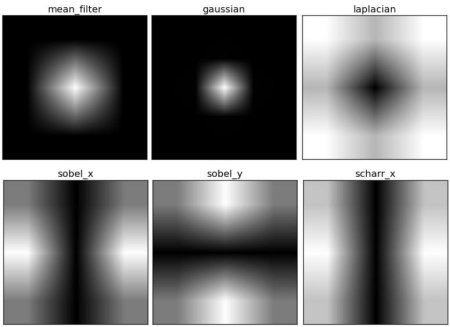展开全文信号处理 opencv 计算机视觉 java
• 我有一个东西，长得特别难看，数学性质特别差，傅立叶变换就是用性质很好的三角函数一部分一部分的去逼近它，最后得出一个看起来很复杂实际上数学性质很好的东西。 代码： # -*- coding: utf-8 -*- import os ...
本质：

就是对信号进行基变换，只不过新的基是正弦/余弦波罢了

我有一个东西，长得特别难看，数学性质特别差，傅立叶变换就是用性质很好的三角函数一部分一部分的去逼近它，最后得出一个看起来很复杂实际上数学性质很好的东西。

代码：

# -*- coding: utf-8 -*-
import os
import numpy as np
import cv2

import numpy as np
from scipy.fftpack import fft, ifft
import matplotlib.pyplot as plt
from matplotlib.pylab import mpl
from mpl_toolkits.mplot3d import Axes3D
import time

mpl.rcParams['font.sans-serif'] = ['SimHei']  # 显示中文
mpl.rcParams['axes.unicode_minus'] = False  # 显示负号

# 采样点选择1400个，因为设置的信号频率分量最高为600赫兹，根据采样定理知采样频率要大于信号频率2倍
a = 0
while a < 100:
a += 1
x = np.linspace(0, 1, 1024)

# 设置需要采样的信号，频率分量有200，400和600
i = np.random.randint(10, size=3)
fr = np.random.randint(512, size=3)

y = i * np.sin(2 * np.pi * fr * x) + i * np.sin(2 * np.pi * fr * x) + i * np.sin(
2 * np.pi * fr * x)

fft_y = fft(y)  # 快速傅里叶变换

N = x.size
x = np.arange(N)  # 频率个数
half_x = x[range(int(N / 2))]  # 取一半区间
time.sleep(1)
abs_y = np.abs(fft_y)  # 取复数的绝对值，即复数的模(双边频谱)
angle_y = np.angle(fft_y)  # 取复数的角度
normalization_y = abs_y / N  # 归一化处理（双边频谱）
normalization_half_y = normalization_y[range(int(N / 2))]  # 由于对称性，只取一半区间（单边频谱）
plt.clf()  # 清除刷新前的图表，防止数据量过大消耗内存
plt.subplot(231)
plt.plot(x, y)
plt.title('原始波形')

plt.subplot(232)
plt.plot(x, fft_y, 'black')
plt.title('双边振幅谱(未求振幅绝对值)', fontsize=9, color='black')

plt.subplot(233)
plt.plot(x, abs_y, 'r')
plt.title('双边振幅谱(未归一化)', fontsize=9, color='red')

plt.subplot(234)
plt.plot(x, angle_y, 'violet')
plt.title('双边相位谱(未归一化)', fontsize=9, color='violet')

plt.subplot(235)
plt.plot(x, normalization_y, 'g')
plt.title('双边振幅谱(归一化)', fontsize=9, color='green')

plt.subplot(236)
plt.plot(half_x, normalization_half_y, 'blue')
plt.title('单边振幅谱(归一化)', fontsize=9, color='blue')
plt.pause(0.4)  # 设置暂停时间，太快图表无法正常显示

plt.ioff()  # 关闭画图的窗口，即关闭交互模式
plt.show()


展开全文• 目标 在本节中，我们将学习 使用OpenCV查找图像的傅立...对于图像，使用2D离散傅里叶变换(DFT)查找频域。一种称为快速傅立叶变换(FFT)的快速算法用于DFT的计算。关于这些的详细信息可以在任何图像处理或信号处理教...
目标
在本节中，我们将学习

使用OpenCV查找图像的傅立叶变换
利用Numpy中可用的FFT函数
傅立叶变换的某些应用程序
我们将看到以下函数：cv.dft()，cv.idft()等

理论
傅立叶变换用于分析各种滤波器的频率特性。对于图像，使用2D离散傅里叶变换(DFT)查找频域。一种称为快速傅立叶变换(FFT)的快速算法用于DFT的计算。关于这些的详细信息可以在任何图像处理或信号处理教科书中找到。请参阅其他资源部分。
对于正弦信号$x(t) = A \sin(2 \pi ft)$，我们可以说$f$是信号的频率，如果采用其频域，则可以看到$f$的尖峰。如果对信号进行采样以形成离散信号，我们将获得相同的频域，但是在$[-π，π]$或$[0,2π]$范围内（对于N点DFT为$[0，N]$）是周期性的。您可以将图像视为在两个方向上采样的信号。因此，在X和Y方向都进行傅立叶变换，可以得到图像的频率表示。
更直观地说，对于正弦信号，如果幅度在短时间内变化如此之快，则可以说它是高频信号。如果变化缓慢，则为低频信号。您可以将相同的想法扩展到图像。图像中的振幅在哪里急剧变化？在边缘点或噪声。因此，可以说边缘和噪声是图像中的高频内容。如果幅度没有太大变化，则它是低频分量。（一些链接已添加到“其他资源”，其中通过示例直观地说明了频率变换）。
现在，我们将看到如何找到傅立叶变换。
Numpy中的傅里叶变换
首先，我们将看到如何使用Numpy查找傅立叶变换。Numpy具有FFT软件包来执行此操作。np.fft.fft2()为我们提供了频率转换，它将是一个复杂的数组。它的第一个参数是输入图像，即灰度图像。第二个参数是可选的，它决定输出数组的大小。如果它大于输入图像的大小，则在计算FFT之前用零填充输入图像。如果小于输入图像，将裁切输入图像。如果未传递任何参数，则输出数组的大小将与输入的大小相同。
现在，一旦获得结果，零频率分量（DC分量）将位于左上角。如果要使其居中，则需要在两个方向上将结果都移动$\frac{N}{2}$。只需通过函数np.fft.fftshift()即可完成。（它更容易分析）。找到频率变换后，就可以找到幅度谱。
import cv2 as cv
import numpy as np
from matplotlib import pyplot as plt
img = cv.imread('messi5.jpg',0)
f = np.fft.fft2(img)
fshift = np.fft.fftshift(f)
magnitude_spectrum = 20*np.log(np.abs(fshift))
plt.subplot(121),plt.imshow(img, cmap = 'gray')
plt.title('Input Image'), plt.xticks([]), plt.yticks([])
plt.subplot(122),plt.imshow(magnitude_spectrum, cmap = 'gray')
plt.title('Magnitude Spectrum'), plt.xticks([]), plt.yticks([])
plt.show()
Result look like below:

结果看起来像下面这样:看，您可以在中心看到更多白色区域，这表明低频内容更多。
因此，您发现了频率变换现在，您可以在频域中进行一些操作，例如高通滤波和重建图像，即找到逆DFT。为此，您只需用尺寸为60x60的矩形窗口遮罩即可消除低频。然后，使用np.fft.ifftshift()应用反向移位，以使DC分量再次出现在左上角。然后使用np.ifft2()函数找到逆FFT。同样，结果将是一个复数。您可以采用其绝对值。
rows, cols = img.shape
crow,ccol = rows//2 , cols//2
fshift[crow-30:crow 31, ccol-30:ccol 31] = 0
f_ishift = np.fft.ifftshift(fshift)
img_back = np.fft.ifft2(f_ishift)
img_back = np.real(img_back)
plt.subplot(131),plt.imshow(img, cmap = 'gray')
plt.title('Input Image'), plt.xticks([]), plt.yticks([])
plt.subplot(132),plt.imshow(img_back, cmap = 'gray')
plt.title('Image after HPF'), plt.xticks([]), plt.yticks([])
plt.subplot(133),plt.imshow(img_back)
plt.title('Result in JET'), plt.xticks([]), plt.yticks([])
plt.show()

结果看起来像下面这样：结果表明高通滤波是边缘检测操作。这就是我们在“图像渐变”一章中看到的。这也表明大多数图像数据都存在于频谱的低频区域。无论如何，我们已经看到了如何在Numpy中找到DFT，IDFT等。现在，让我们看看如何在OpenCV中进行操作。
如果您仔细观察结果，尤其是最后一张JET颜色的图像，您会看到一些伪像（我用红色箭头标记的一个实例）。它在那里显示出一些波纹状结构，称为振铃效应。这是由我们用于遮罩的矩形窗口引起的。此掩码转换为正弦形状，从而导致此问题。因此，矩形窗口不用于过滤。更好的选择是高斯窗口。
OpenCV中的傅里叶变换
OpenCV为此提供了cv.dft()和cv.idft()函数。它返回与前一个相同的结果，但是有两个通道。第一个通道是结果的实部，第二个通道是结果的虚部。输入图像首先应转换为np.float32。我们来看看怎么做。
import numpy as np
import cv2 as cv
from matplotlib import pyplot as plt
img = cv.imread('messi5.jpg',0)
dft = cv.dft(np.float32(img),flags = cv.DFT_COMPLEX_OUTPUT)
dft_shift = np.fft.fftshift(dft)
magnitude_spectrum = 20*np.log(cv.magnitude(dft_shift[:,:,0],dft_shift[:,:,1]))
plt.subplot(121),plt.imshow(img, cmap = 'gray')
plt.title('Input Image'), plt.xticks([]), plt.yticks([])
plt.subplot(122),plt.imshow(magnitude_spectrum, cmap = 'gray')
plt.title('Magnitude Spectrum'), plt.xticks([]), plt.yticks([])
plt.show()


注意
您还可以使用cv.cartToPolar()，它在单个镜头中同时返回幅值和相位

现在我们要做DFT的逆变换。在上一节中，我们创建了一个HPF，这次我们将看到如何删除图像中的高频内容，即我们将LPF应用到图像中。它实际上模糊了图像。为此，我们首先创建一个高值(1)在低频部分，即我们过滤低频内容，0在高频区。
rows, cols = img.shape
crow,ccol = rows/2 , cols/2
# 首先创建一个掩码，中心正方形为1，其余全为零
mask = np.zeros((rows,cols,2),np.uint8)
mask[crow-30:crow 30, ccol-30:ccol 30] = 1
# 应用掩码和逆DFT
fshift = dft_shift*mask
f_ishift = np.fft.ifftshift(fshift)
img_back = cv.idft(f_ishift)
img_back = cv.magnitude(img_back[:,:,0],img_back[:,:,1])
plt.subplot(121),plt.imshow(img, cmap = 'gray')
plt.title('Input Image'), plt.xticks([]), plt.yticks([])
plt.subplot(122),plt.imshow(img_back, cmap = 'gray')
plt.title('Magnitude Spectrum'), plt.xticks([]), plt.yticks([])
plt.show()

看看结果：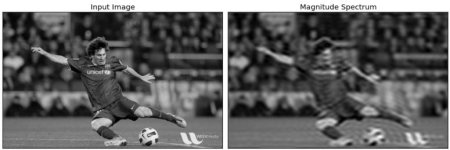注意
通常，OpenCV函数cv.dft()和cv.idft()比Numpy函数更快。但是Numpy函数更容易使用。有关性能问题的更多细节，请参见下面的部分。

DFT的性能优化
对于某些数组尺寸，DFT的计算性能较好。当数组大小为2的幂时，速度最快。对于大小为2、3和5的乘积的数组，也可以非常有效地进行处理。因此，如果您担心代码的性能，可以在找到DFT之前将数组的大小修改为任何最佳大小(通过填充零)。对于OpenCV，您必须手动填充零。但是对于Numpy，您指定FFT计算的新大小，它将自动为您填充零。
那么如何找到最优的大小呢?OpenCV为此提供了一个函数，cv.getOptimalDFTSize()。它同时适用于cv.dft()和np.fft.fft2()。让我们使用IPython魔术命令timeit来检查它们的性能。
In : img = cv.imread('messi5.jpg',0)
In : rows,cols = img.shape
In : print("{} {}".format(rows,cols))
342 548
In : nrows = cv.getOptimalDFTSize(rows)
In : ncols = cv.getOptimalDFTSize(cols)
In : print("{} {}".format(nrows,ncols))
360 576

参见，将大小(342,548)修改为(360，576)。现在让我们用零填充（对于OpenCV），并找到其DFT计算性能。您可以通过创建一个新的零数组并将数据复制到其中来完成此操作，或者使用cv.copyMakeBorder()。
nimg = np.zeros((nrows,ncols))
nimg[:rows,:cols] = img

或者:
right = ncols - cols
bottom = nrows - rows
bordertype = cv.BORDER_CONSTANT ＃只是为了避免PDF文件中的行中断
nimg = cv.copyMakeBorder(img,0,bottom,0,right,bordertype, value = 0)

现在，我们计算Numpy函数的DFT性能比较：
In : %timeit fft1 = np.fft.fft2(img)
10 loops, best of 3: 40.9 ms per loop
In : %timeit fft2 = np.fft.fft2(img,[nrows,ncols])
100 loops, best of 3: 10.4 ms per loop

它显示了4倍的加速。现在，我们将尝试使用OpenCV函数。
In : %timeit dft1= cv.dft(np.float32(img),flags=cv.DFT_COMPLEX_OUTPUT)
100 loops, best of 3: 13.5 ms per loop
In : %timeit dft2= cv.dft(np.float32(nimg),flags=cv.DFT_COMPLEX_OUTPUT)
100 loops, best of 3: 3.11 ms per loop

它还显示了4倍的加速。您还可以看到OpenCV函数比Numpy函数快3倍左右。也可以对逆FFT进行测试，这留给您练习。
为什么拉普拉斯算子是高通滤波器？
在一个论坛上也有人提出了类似的问题。问题是，为什么拉普拉斯变换是高通滤波器?为什么Sobel是HPF?等。第一个答案是关于傅里叶变换的。对于更大的FFT只需要拉普拉斯变换。分析下面的代码：
import cv2 as cv
import numpy as np
from matplotlib import pyplot as plt
# 没有缩放参数的简单均值滤波器
mean_filter = np.ones((3,3))
# 创建高斯滤波器
x = cv.getGaussianKernel(5,10)
gaussian = x*x.T
# 不同的边缘检测滤波器
# x方向上的scharr
scharr = np.array([[-3, 0, 3],
[-10,0,10],
[-3, 0, 3]])
# x方向上的sobel
sobel_x= np.array([[-1, 0, 1],
[-2, 0, 2],
[-1, 0, 1]])
# y方向上的sobel
sobel_y= np.array([[-1,-2,-1],
[0, 0, 0],
[1, 2, 1]])
# 拉普拉斯变换
laplacian=np.array([[0, 1, 0],
[1,-4, 1],
[0, 1, 0]])
filters = [mean_filter, gaussian, laplacian, sobel_x, sobel_y, scharr]
filter_name = ['mean_filter', 'gaussian','laplacian', 'sobel_x', \
'sobel_y', 'scharr_x']
fft_filters = [np.fft.fft2(x) for x in filters]
fft_shift = [np.fft.fftshift(y) for y in fft_filters]
mag_spectrum = [np.log(np.abs(z) 1) for z in fft_shift]
for i in xrange(6):
plt.subplot(2,3,i 1),plt.imshow(mag_spectrum[i],cmap = 'gray')
plt.title(filter_name[i]), plt.xticks([]), plt.yticks([])
plt.show()

看看结果：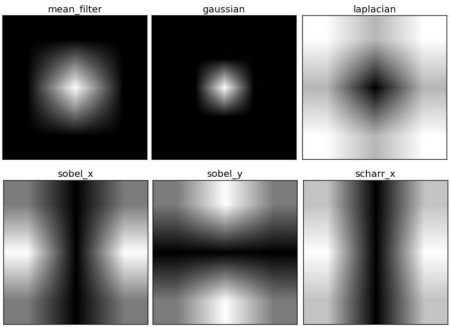从图像中，您可以看到每种内核阻止的频率区域以及它允许经过的区域。从这些信息中，我们可以说出为什么每个内核都是HPF或LPF
附加资源
1.傅里叶变换的直观解释：http://cns-alumni.bu.edu/~slehar/fourier/fourier.html by Steven Lehar
2. 傅里叶变换：http://homepages.inf.ed.ac.uk/rbf/HIPR2/fourier.htm at HIPR
3. 图像中的频率域指什么？http://dsp.stackexchange.com/q/1637/818
欢迎关注磐创博客资源汇总站：
http://docs.panchuang.net/
欢迎关注PyTorch官方中文教程站：
http://pytorch.panchuang.net/
OpenCV中文官方文档：
http://woshicver.com/


展开全文• 傅里叶变换及高通/低通滤波目标前言1. Numpy中的傅里叶变换2. OpenCV中的傅里叶变换3. 为什么拉普拉斯算子是高通滤波器？附加资源 目标 通过本篇文章的学习，你将学习到以下内容： 使用OpenCV查找图像的傅立叶变换 ...
傅里叶变换及高通/低通滤波目标前言1. Numpy中的傅里叶变换2. OpenCV中的傅里叶变换3. 为什么拉普拉斯算子是高通滤波器？4. 总结附加资源
目标
通过本篇文章的学习，你将学习到以下内容：

使用OpenCV查找图像的傅立叶变换
利用Numpy中可用的FFT函数
傅立叶变换的某些应用
学习到函数：cv.dft()，cv.idft()

前言
傅立叶变换用于分析各种滤波器的频率特性。对于图像，使用2D离散傅里叶变换(DFT)查找频域。一种称为快速傅立叶变换(FFT)的快速算法用于DFT的计算。
对于正弦信号$x(t) = A \sin(2 \pi ft)$，我们可以说$f$是信号的频率，如果采用其频域，则可以看到$f$的尖峰。如果对信号进行采样以形成离散信号，我们将获相同的频域，但是在[-π，π]或[0,2π]范围内（对于N点DFT为[0，N]）是周期性的，你可以将图像视为在两个方向上采样的信号。因此，在X 和Y方向都进行傅立叶变换，可以得到图像的频率表示。
更直观地说，对于正弦信号，如果幅度在短时间内变化如此之快，则可以说它是高频信号。如果变化缓慢，则为低频信号。你可以将相同的方式扩展到图像。图像中的振幅在哪里急剧变化？在边缘点或噪声。因此，可以说边缘和噪声是图像中的高频内容。如果幅度没有太大变化，则它是低频分量。（一些链接已添加到“其他资源”，其中通过示例直观地说明了频率变换）。
接下来，我们将学习如何找到傅立叶变换。
1. Numpy中的傅里叶变换
首先，我们将看到如何使用Numpy查找傅立叶变换。Numpy具有FFT软件包来执行此操作。np.fft.fft2()为我们提供了频率转换，它将是一个复杂的数组。它的第一个参数是输入图像，即灰度图像。第二个参数是可选的，它决定输出数组的大小。如果它大于输入图像的大小，则在计算FFT之前用零填充输入图像。如果小于输入图像，将裁切输入图像。如果未传递任何参数，则输出数组的大小将与输入的大小相同。
现在，一旦获得结果，零频率分量（DC分量）将位于左上角。如果要使其居中，则需要在两个方向上将结果都移动$\frac{N}{2}$。只需通过函数np.fft.fftshift()即可完成。（它更容易分析）。找到频率变换后，就可以找到幅度谱。
import cv2 as cv
import numpy as np
from matplotlib import pyplot as plt

img = cv.imread('./data/messi5.jpg', 0)
f = np.fft.fft2(img)
fshift = np.fft.fftshift(f)
magnitude_spectrum = 20*np.log(np.abs(fshift))

plt.subplot(121), plt.imshow(img, 'gray'), plt.title('Input image')
plt.xticks([]), plt.yticks([])
plt.subplot(122), plt.imshow(magnitude_spectrum, 'gray'), plt.title('Magnitude spectrum')
plt.xticks([]), plt.yticks([])
plt.show()从图中你可以在中心处看到更多白色区域，这表明低频内容更多。
实现了频率变换后，你可以在频域中进行一些操作，例如高通滤波和重建图像，即找到逆DFT。为此，你只需用尺寸为60x60的矩形窗口遮罩即可消除低频。然后，使用np.fft.ifftshift()应用反向移位，以使DC分量再次出现在左上角。然后使用np.fft.ifft2()函数找到逆FFT。同样，结果将是一个复数。你可以采用其绝对值。
import cv2 as cv
import numpy as np
from matplotlib import pyplot as plt

img = cv.imread('./data/messi5.jpg', 0)
f = np.fft.fft2(img)
fshift = np.fft.fftshift(f)

rows, cols = img.shape
crow, ccol = rows//2, cols//2
fshift[crow-30:crow+31, ccol-30:ccol+31] = 0
f_ishift = np.fft.ifftshift(fshift)
img_back = np.fft.ifft2(f_ishift)
img_back = np.real(img_back)

plt.subplot(131), plt.imshow(img, 'gray')
plt.title('Input image'), plt.xticks([]), plt.yticks([])
plt.subplot(132), plt.imshow(img_back, 'gray')
plt.title('Image after HPF'), plt.xticks([]), plt.yticks([])
plt.subplot(133), plt.imshow(img_back)
plt.title('Result in JET'), plt.xticks([]), plt.yticks([])
plt.show()结果表明高通滤波是边缘检测操作。这就是我们在“图像渐变”一章中看到的。这也表明大多数图像数据都存在于频谱的低频区域。无论如何，我们已经看到了如何在Numpy中找到DFT，IDFT等。现在，让我们看看如何在OpenCV中进行操作。 如果您仔细观察结果，尤其是最后一张JET颜色的图像，您会看到一些伪像（我用红色箭头标记的一个实例）。它在那里显示出一些波纹状结构，称为振铃效应。这是由我们用于遮罩的矩形窗口引起的。此掩码转换为正弦形状，从而导致此问题。因此，矩形窗口不用于过滤。更好的选择是高斯窗口。
2. OpenCV中的傅里叶变换
OpenCV为此提供了cv.dft()和cv.idft()函数。它返回与前一个相同的结果，但是有两个通道。第一个通道是结果的实部，第二个通道是结果的虚部。输入图像首先应转换为np.float32 。我们来看看怎么做。
import numpy as np
import cv2 as cv
from matplotlib import pyplot as plt
img = cv.imread('./data/messi5.jpg',0)
dft = cv.dft(np.float32(img),flags = cv.DFT_COMPLEX_OUTPUT)
dft_shift = np.fft.fftshift(dft)
magnitude_spectrum = 20*np.log(cv.magnitude(dft_shift[:,:,0],dft_shift[:,:,1]))

plt.subplot(121),plt.imshow(img, cmap = 'gray')
plt.title('Input Image'), plt.xticks([]), plt.yticks([])
plt.subplot(122),plt.imshow(magnitude_spectrum, cmap = 'gray')
plt.title('Magnitude Spectrum'), plt.xticks([]), plt.yticks([])
plt.show()注意： 您还可以使用cv.cartToPolar()，它在单个镜头中同时返回幅值和相位

现在我们要做DFT的逆变换。在上一节中，我们创建了一个HPF，这次我们将看到如何删除图像中的高频内容，即我们将LPF应用到图像中。它实际上模糊了图像。为此，我们首先创建一个高值1在低频部分，即我们过滤低频内容，0在高频区。
import numpy as np
import cv2 as cv
from matplotlib import pyplot as plt
img = cv.imread('./data/messi5.jpg',0)
dft = cv.dft(np.float32(img),flags = cv.DFT_COMPLEX_OUTPUT)
dft_shift = np.fft.fftshift(dft)

rows, cols = img.shape
crow,ccol = rows//2 , cols//2
# 首先创建一个掩码，中心正方形为1，其余全为零
mask = np.zeros((rows,cols,2),np.uint8)
mask[crow-30:crow+30, ccol-30:ccol+30] = 1
# 应用掩码和逆DFT
fshift = dft_shift*mask
f_ishift = np.fft.ifftshift(fshift)
img_back = cv.idft(f_ishift)
img_back = cv.magnitude(img_back[:,:,0],img_back[:,:,1])
plt.subplot(121),plt.imshow(img, cmap = 'gray')
plt.title('Input Image'), plt.xticks([]), plt.yticks([])
plt.subplot(122),plt.imshow(img_back, cmap = 'gray')
plt.title('Magnitude Spectrum'), plt.xticks([]), plt.yticks([])
plt.show()注意： 通常，OpenCV函数cv.dft()和cv.idft()比Numpy函数更快。但是Numpy函数更容易使用。

3. 为什么拉普拉斯算子是高通滤波器？
在一个论坛上也有人提出了类似的问题。问题是，为什么拉普拉斯变换是高通滤波器?为什么Sobel是HPF?等。第一个答案是关于傅里叶变换的。对于更大的FFT只需要拉普拉斯变换。分析下面的代码：
import cv2 as cv
import numpy as np
from matplotlib import pyplot as plt
# 没有缩放参数的简单均值滤波器
mean_filter = np.ones((3,3))
# 创建高斯滤波器
x = cv.getGaussianKernel(5,10)
gaussian = x*x.T
# 不同的边缘检测滤波器
# x方向上的scharr
scharr = np.array([[-3, 0, 3],
[-10,0,10],
[-3, 0, 3]])
# x方向上的sobel
sobel_x= np.array([[-1, 0, 1],
[-2, 0, 2],
[-1, 0, 1]])
# y方向上的sobel
sobel_y= np.array([[-1,-2,-1],
[0, 0, 0],
[1, 2, 1]])
# 拉普拉斯变换
laplacian=np.array([[0, 1, 0],
[1,-4, 1],
[0, 1, 0]])
filters = [mean_filter, gaussian, laplacian, sobel_x, sobel_y, scharr]
filter_name = ['mean_filter', 'gaussian','laplacian', 'sobel_x', \
'sobel_y', 'scharr_x']
fft_filters = [np.fft.fft2(x) for x in filters]
fft_shift = [np.fft.fftshift(y) for y in fft_filters]
mag_spectrum = [np.log(np.abs(z)+1) for z in fft_shift]
for i in range(6):
plt.subplot(2, 3, i + 1), plt.imshow(mag_spectrum[i], cmap='gray')
plt.title(filter_name[i]), plt.xticks([]), plt.yticks([])
plt.show()从图像中，你可以看到每种内核阻止的频率区域以及它允许经过的区域。从这些信息中，我们可以说出为什么每个内核都是HPF或LPF。
4. 总结
本篇文章介绍了图像处理中的傅里叶变换，主要介绍了Numpy中的傅里叶变换及其逆变换，OpenCV中的傅里叶变换及其逆变换，还解释了为什么拉普拉斯算子是高通滤波器的原因。
附加资源

傅里叶变换的直观解释：http://cns-alumni.bu.edu/~slehar/fourier/fourier.html by Steven Lehar
傅里叶变换：添加链接描述  at HIPR
图像中的频率域指什么？http://dsp.stackexchange.com/q/1637/818

如果文章对你有帮助，欢迎一键三连哦~~， 谢谢~~


展开全文opencv 计算机视觉
• 文章目录直接上代码主文件a9.py画多图的函数写在一个新的文件a9mysubplot里：将a9.py和a9mysubplot.py放在同一个文件夹里，运行a9.py文件结果显示原始信号原始信号中的正弦信号的频谱以(5,5)权重、(3,7)权重...
• 使用python进行傅里叶变换 实验目的 1.掌握使用matplotlib进行绘图的基本步骤 2. 利用python程序实现傅里叶变换 实验原理 傅立叶变换是一种分析信号的方法，它可分析信号的成分，也可用这些成分合成信号。许多波形可...可视化
• 文章目录python语音信号快速傅里叶变换快速傅里叶变换的原理引入必要的库快速傅里叶变换函数用法 快速傅里叶变换的原理 引入必要的库 import numpy as np import matplotlib.pyplot as plt from scipy.fftpack ...
• python numpy 傅里叶变换与频域图 """ 三角函数 合成方波 傅里叶变换与逆傅里叶变换 绘制频域图 """ import numpy as np import matplotlib.pyplot as mp import numpy.fft as nf # 准备x值与y值 x = np....
• 可实现对一些基本的信号波形以及自定义波形进行傅里叶变换，并以图形形 式显示结果。基本信号波形至少包括冲激函数、门函数、正/余弦函数，并支持 设置必要的参数，如门函数的时间宽度、正/余弦函数的频率等，自定义...
• 对于一维的连续函数，定义域为整个时间轴的非周期函数f(t)，它的傅里叶变换为 对应的逆傅里叶变换为 一维的离散函数 (其中x=0，1，2，...，M-1) 的傅里叶变换和逆变换为 对于二维的情况，二维连续...
• 内容来自OpenCV-Python Tutorials 自己翻译整理 傅里叶变换原理的文章 目标 用opencv对图像进行傅里叶变换 numpy进行快速傅里叶变换 傅里叶变换的用处 学习函数函数cv2.dft()，cv2.idft()opencv
• OpenCV提供了函数cv2.dft()和cv2.idft()来实现傅里叶变换和逆傅里叶变换，下面分别展开介绍。 14.3.1 实现傅里叶变换 函数cv2.dft()的语法格式为： 返回结果=cv2.dft(原始图像，转换标识) 在使用该函数时，需要...
• OpenCV为此提供了cv.dft()和cv.idft()函数...傅里叶变换 import cv2 import numpy as np import matplotlib.pyplot as plt img = cv2.imread('images/lena.jpg',0) img_float32 = np.float32(img) cv2.imshow("img",imopencv
• 码字不易，如果对您有所帮助，记着点赞哦！... 对二维图像进行傅里叶变换用如下公式进行： 图像长M，高N。F(u,v)表示频域图像，f(x,y)表示时域图像。u的范围为[0,M-1]，v的范围为[0,N-1] ↑ 对二维图...数字图像处理
• 数值计算-利用Python实现快速傅里叶变换(FFT)(不调用函数库) 快速傅里叶变换介绍 首先介绍离散傅里叶变换（DFT），不管是正变换还是逆变换，计算公式可以归结为 X(k)=∑n=0N−1x(n)WNnk X(k)=\sum_{n=0}^{N-1}x(n)W_...numpy
• 一、前言  傅里叶变换相关函数  基于傅里叶变换的频域滤波  离散傅里叶变换（DFT）二、短时傅里叶变换stft三、frequency bin参考 一、前言 　我想认真写好快速傅里叶变换（Fast Fourier Transform，FFT），...信号处理 数据分析
• 目录二变量函数傅里叶变换二维冲激及其取样性质二维连续傅里叶变换对二维取样和二维取样定理图像中的混叠二维离散傅里叶变换及其反变换 二变量函数傅里叶变换 二维冲激及其取样性质 两个连续变量的冲激函数定义...图像处理 numpy opencv
• 摘要：一直以来都是用MATLAB做信号处理，得到预处理的特征后再用Python进一步应用神经网络之类的方法。这里将MATLAB中的FFT、STFT、加窗以及带通滤波通过Python接口实现，防止以后MATLAB用不了了，一定程度上也提高...信号处理 滤波
• ## 常用函数的傅里叶变换汇总

万次阅读 多人点赞 2020-03-19 12:42:42
快速链接: ...常用函数傅里叶变换汇总 (3) LTI 系统 与 滤波器 常用函数傅里叶变换汇总 f(t)⟵⟶F(jω)F(jt)⟵⟶2πf(−ω)f(αt)⟵⟶1∣α∣F(jωα)a⋅f1+b⋅f2⟵⟶a⋅F1+b⋅F2f(t±...信号处理
• Numpy模块提供了傅里叶变换功能，Numpy模块中的fft2()函数可以实现图像的傅里叶变换。本节介绍如何用Numpy模块实现图像的傅里叶变换，以及在频域内过滤图像的低频信息，保留高频信息，实现高通滤波。 14.2.1 实现...图像处理
• python-opencv(13)傅里叶变换 文章目录1. 傅里叶变换的理论基础2. numpy实现傅里叶变换2.1. 相关的函数2.1 代码实现3. numpy实现逆傅里叶变换3.1 相关的函数3.2 代码实现4. 在频域进行高通滤波4.1 对高频、低频和...
•  相比于MATLAB自带的FFT函数以及详尽的官方文档来说，python傅里叶变换这个方面相比就不是那么简单了，处处需要使用Help查看相关函数的定义。但是本质来说，都是傅里叶变换，只是编程语言不同而已。 一、使用到......

# python傅里叶变换函数python 订阅